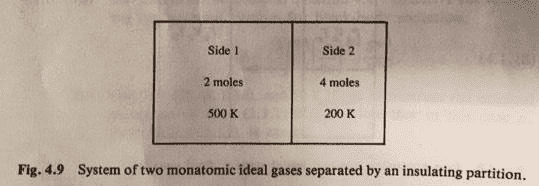# Determining the change of entropy

## Homework Statement

A composite system consists of an insulated partitioned volume, shown in Figure 4.9. Side 1 contains 2 mol of a monatomic ideal gas and Side 2 contains 4 moles of the same monatomic ideal gas. Initially, the two sides are separated by a fully insulated partition (adiabatic wall) and the temperatures are 500K and 200K on sides 1 and 2 respectively. At a particular time replacing the partition by a conducting frictionless piston. Initially V1=10L and V2=5L . Let T12 be the common temperature at which equilibrium is reached and P12 the common pressure.a.) Find T12 (Found to be 300K)
b.) Find P12, and the new values for V1 and V2. (Found them to be 998kPa, 5L and 10L respectively)
c.) Determine ΔS, as in ΔS=ΔS1+ΔS2 (The answer is 19J/K, but I cannot seem to reach that answer)

## Homework Equations

ΔS=Ncv ln(T/To) + NR ln (V/Vo)

## The Attempt at a Solution

So I found ΔS1= -17.896 using T=300, To=500, V=5, Vo=10 And ΔS2= 43.278 with T=300, To=200, V=10, Vo=5. I used cv=3/2R (though I thought it should be 3/2NR, but in class we had that it should be 3/2R for this question). So these values give a ΔS of 25.382J/K, which is quite a bit off from the answer. Any help would be greatly appreciated.